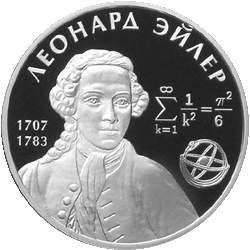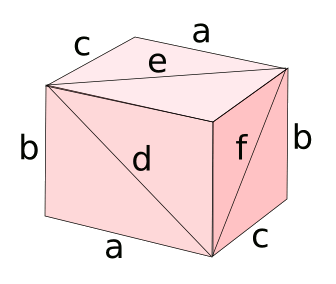### Euler Bricks

April 26, 2013

You can likely gauge the mathematical precocity of a child by the age at which he or she exhibits knowledge of one of the many things associated with Leonhard Euler. Euler accomplished much in his life, and he was not just an eminent mathematician, but a physicist who made contributions to mechanics and astronomy. A principal scientific contribution was his development of the Huygens wave theory of light as a counterpoint to the corpuscular theory in Newton's Opticks.A Russian two-ruble coin celebrating the 300th anniversary of Euler's birth.

The equation is Euler's identification of the Basel problem as an infinite series formula of pi.

(Via Wikimedia Commons))

Finding interesting mathematics problems was relatively easy in Euler's day, since there was so much mathematics undiscovered. You can imagine Euler taking a stroll to the market, perhaps to buy more ink, since he had just penned another lengthy treatise. He drops a coin on the roadway, and he contemplates a road brick as he stoops to pick it up. At that point he imagines what's now called an Euler brick.[1-3]

An Euler brick is a rectangular cuboid whose edges and face diagonals all have integer length. The edges and diagonal of any face of an Euler brick will be a Pythagorean triple, and there are many integer sets that comprise a Pythagorean triple. The number of sets of edge lengths accessible to an Euler brick are far less, since they must satisfy the following equations simultaneously.
a2 + b2 = d2
a2 + c2 = e2
b2 + c2 = f2
in which a, b and c are the lengths of the sides, and d, e and f are the diagonals, as shown in the figure. These must all be integers.Diagram of an Euler brick.

(Created by the author using Inkscape)

Not surprisingly, The On-Line Encyclopedia of Integer Sequences™ has three sequences associated with Euler bricks. These are the lengths of the shortest edge, the intermediate edge, and the longest edge. We can imagine a more perfect Euler brick for which the space diagonal (any one of the four equivalent diagonals criss-crossing the interior space) is also an integer, but no example of such a perfect cuboid has been found. Computer searches up to a smallest edge of 1010 have been unsuccessful.

There are ten Euler bricks in the range (1 ≤ a ≤ b ≤ c ≤ 1000). These are listed in the table below.

 a b c a b c 44 117 240 160 231 792 85 132 720 176 468 960 88 234 480 240 252 275 132 351 720 480 504 550 140 480 693 720 756 825

Since I needed some coding practice, I wrote a program to find the dimensions of Euler bricks. You can find the source code, albeit in my far too casual coding style, here. The program works well for small numbers, and it wouldn't be too hard to modify it for big numbers through use of an arbitrary-precision arithmetic library, such as the GNU Multiple Precision Arithmetic Library.

In the introduction, I wrote about mathematics being "discovered." There's a question as to whether mathematics is invented or discovered. As I explained in a previous article (Mathematical Objects, May 9, 2008), I'm a member of the discovery camp, in whose company we find Plato, and our contemporary mathematician, Roger Penrose.

### References:

Linked Keywords: Mathematics; mathematical; Leonhard Euler; mathematician; physicist; mechanics; astronomy; Christiaan Huygens; wave theory of light; corpuscular theory; Isaac Newton; Opticks; Basel problem; infinite series formula of pi; Wikimedia Commons; brick; Euler brick; rectangle; rectangular; cuboid; edge; diagonal; integer; Pythagorean triple; set; equation; Inkscape; The On-Line Encyclopedia of Integer Sequences™; shortest edge; intermediate edge; longest edge; brute-force search; coding; computer program; source code; arbitrary-precision arithmetic library; GNU Multiple Precision Arithmetic Library; Plato; Roger Penrose.